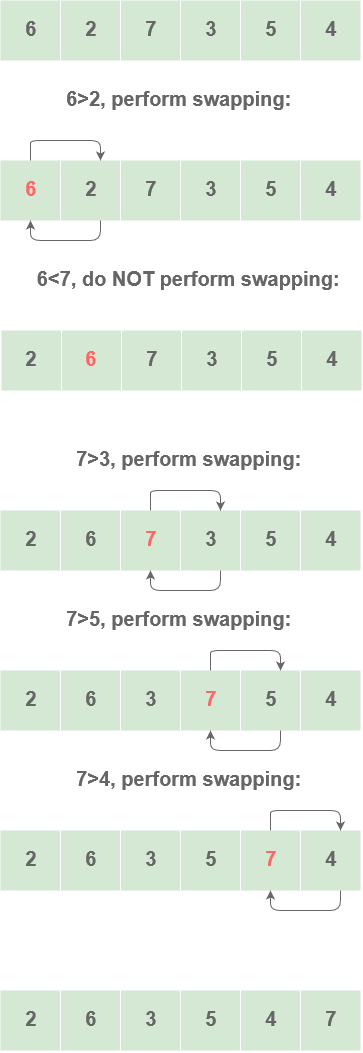# Bubble Sort

## Bubble Sort Algorithm

Bubble sort is an algorithm in which all the elements are traversed one by one and compared with the adjacent element and then swapped according to the order specified. The order can be ascending or descending.
All the elements are compared one by one and sorted based on their values.

For an array of size `N`, the process has to be repeated `N-1` times.

## Implementation

Steps

• Starting with the first element, that is the first index
• Compare the first element with the next element in the array.
• If the first element is greater than the second element, swap them.
• If the above condition fails, move to the next element.
• Now, compare the second and third elements. If they are not in order, swap them
• Continue the above process until the last element.

`Taking the array : {6, 2, 7, 3, 4, 3}`

First iteration of bubble sort is shown :Perform the same process for the remaining iterations.
At the end of each iteration, the largest element gets placed at the end.
In each iteration, the comparison takes place up to the last element.

Code

``````void BubbleSort(int A[],int size)
{   int i,j,temp;
for (i=0;i<size;i++)
{
for (j=0;j<size-i-1;j++)
{
if(A[j]>A[j+1])
{
temp = A[j];
A[j]= A[j+1];
A[j+1]= temp;
}
}
}
}
``````

#### Complexity: O(n^2)

Since there are 2 loops running for O(n) time, the complexity is O(n)*O(n) = O(n^2)

Scroll to Top
[gravityforms id="5" description="false" titla="false" ajax="true"]